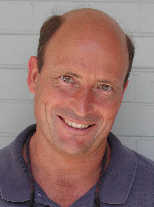### Math 3150 Coordinating Page

Nick Korevaar's home page
Department of Mathematics
College of Science
University of UtahThese notes and links are on-line, at the URL http://www.math.utah.edu/~korevaar/coord3150. Please contact me, Nick Korevaar, if you have any questions about teaching this class. My contact information is on my home page http://www.math.utah.edu/~korevaar.

Every instructor should be familiar with and follow the advice in the Departmental teaching guide.

Math 3150 is a 2-credit hour, basic introduction to partial differential equations in two or fewer space variables. No previous exposure to partial differential equations is assumed. However, it is assumed that students know certain concepts from calculus, ordinary differential equations and linear algebra: The formal prerequisites are grades of at least "C" in single and multivariable calculus (i.e. through Math 2210, 1280, or 1260); and linear algebra and differential equations, Math 2250 or 2270-2280.

After an introductory chapter about the origins, uses and study of partial differential equations, there is a careful discussion of Fourier series for periodic functions. The course focuses on the wave equation, heat equation, and Laplace's equation, and uses separation of variables on rectangular and circular domain geometries. Bessel's functions are introduced as appropriate to understand separated solutions on circular domains. The Fourier transform is introduced for infinite spatial domains.

You'll notice that the synopsis above does not exactly line up with the one in the official UU Math catalog: http://www.acs.utah.edu/GenCatalog/crsdesc/math.html

This course mainly serves engineering and physics majors. Math majors also sometimes take 3150, even though it does not count for the regular Math major. (Usually, Math 2280 also covers a fair portion of this material.) This is a math course, and so it is important to motivate and explain why the math works. However, this is primarily a service course for other departments. Since most of the students are actually in allied fields they will especially appreciate examples which show applications, and a focus on problem solving as opposed to theorem proving. While still explaining where ideas come from and why they work, do tone down the rigor and emphasize problem solving, as compared to what you would do for an audience of Math majors.

Homework should be assigned, collected and graded. Contact Angie Gardiner (gardiner"at"math.utah.edu) for a grader as early as possible. There should be several in-class midterms, and an in-class final exam. It is natural to use computer software to enhance this course material, and Maple (alternately Matlab) problems, demonstrations, or projects are very appropriate. Students should already be at least passively familiar with Maple, as it is used in Math 2250, 2270-2280.

Textbook: Partial Differential Equations with Fourier Series and Boundary Value Problems, second edition, by Nakhle Asmar. The current ISBN is 9780131480964. The author provides a link to a student solutions manual (with solutions to problem numbers 4n+1, n a whole number), along with other resources, at his home page http://www.math.missouri.edu/~nakhle

The sections to be covered are 1.1-1.2; 2.1-2.4; 3.1-3.9; 4.1-4.4; 7.1-7.4. Instructors who have been pressed for time have sometimes omitted 4.3-4.4 and 7.3-7.4. Alternately, some have managed to cover almost all of the core, along with 4.7-4.8, 7.8-7.9. You will typically have 28-29 contact days if you are teaching fall or spring semester. Allowing for a couple of exam days and potential review/catch-up time, you will need to plan carefully in order to cover all the material.

One possibility to consider, and a strategy I follow in many of my classes, is to schedule one of your office hours in a classroom, and call it an optional problem session. This will encourage students to show up, and you can give help on how to work the homework problems and augment your classroom explanations. If you follow this strategy, either let your students know the time of this optional session as early as possible, or pick the time in consultation with them, during the first day of class; even before the start of fall term you can take advantage of the CIS capability which lets you send emails to your class.

Previous home pages: Recent instructors of Math 3150 here at Utah include Spencer Stirling, Sasha Balk, Michael van Opstall and Jingyi Zhu, among others. Previous instructors can be excellent resources if you have questions about teaching this class. Here are links to their home pages or course syllabi for Math 3150; these may be very helpful as you construct and teach your own course:
http://www.math.utah.edu/~opstall/3150
http://www.spencerstirling.com/teaching/M3150/M3150.html
http://www.math.utah.edu/~zhu/3150.html
http://www.math.utah.edu/~balk/3150syllabus.pdf